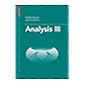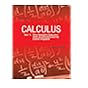Normal view MARC view ISBD view

# Calculus, vol. I One-Variable Calculus, with an introduction to linear algebra

Material type:TextPublisher: New York, John Wiley 2002Edition: 2nd ed.Description: 666 p. illus.ISBN: 997151396X.Subject(s): Mathematical analysis | CalculusSummary: (Acc. No. 2568) Instructors Copy
List(s) this item appears in: Reserved for the semester Aug-Nov 2017
Item type Current location Call number Status Date due Barcode Item holds
Book Chennai Mathematical Institute
515 APO (Browse shelf) Available 2568
Book Chennai Mathematical Institute
General Stacks
515 APO (Browse shelf) Available 2877
Total holds: 0
##### Browsing Chennai Mathematical Institute Shelves , Shelving location: General Stacks Close shelf browser
 No cover image available No cover image available No cover image available No cover image available515 AMA Analysis I / 515 AMA Analysis II / 515 AMA Analysis III / 515 APO Calculus, vol. I One-Variable Calculus, with an introduction to linear algebra 515 APO Calculus, vol. II Multi-Variable Calculus and LInear Algebra, with Applications to Differential Equations and Probability 515 APO Calculus / Vol. 1 one variable calculus, with an introduction to linear algebra. 515 APO Calculus / Vol. 1 one variable calculus, with an introduction to linear algebra.

(Acc. No. 2568) Instructors Copy